Next: Analyzing systems of equations Up: Solving with Interval Analysis Previous: Initial domain and simplification   Contents

Filtering a system of equation

As mentioned previously the 2B heuristic to improve the solving is to rewrite each equation as the equality of two different terms, to determine if the interval evaluation of both terms are consistent and if not to adjust the interval for one term and by using the inverse function for this term to improve the width for one or more unknowns.

For example imagine that one of the equation is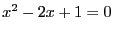. The procedure will introduce a new variable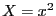such that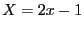and compute its interval evaluation. If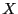has a negative upper bound the equation has no solution for the current range for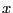. If the upper bound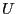ofis positive then the inverse function ofindicates thatshould lie in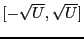: we may then update the interval forif this is not the case. If the lower bound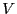ofis positive then the inverse function ofindicates thatshould lie outside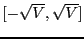. If the range foris included in this interval, then there is no solution to the equation for this range for.

We have already seen that the procedure HullConsistency of ALIAS-Maple implements this consistency. But this is only a partial implementation as only linear and square terms in the unknowns are considered. A more general implementation has been written by G. Chabert.

To use this implementation you need to prepare first a file such as:

size : 1 2
vars : x y
constraints :
x*y^2-x+y-sin(x)-3=0
size is followed by the number of equations and number of variables, vars indicate the unknown names and constraints is followed by the equations which are written using a standard mathematical notation.

This description of the system is loaded using the C++ procedure load_equation. This procedure has an optional second argument which is a floating point number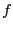whose use will be described later on.

The 2B procedure is then used by calling the C++ procedure _2b(P), where P is an interval vector which defines the range for each unknown. If this procedure returns -1, then the equations have no solution in P, otherwise P may have been improved (i.e. one or more of the ranges of P may have now a smaller diameter).

The role ofin load_equation is that as soon as a range for one (or more) unknown has a diameter that has been decreased by more thancompared to its initial value, then the 2B procedure will be repeated for all equations and all unknowns.

Note:

• the _2b procedure allows to deal with a large number of operators but may miss some special cases. If a constraint is not recognized load_equation will create a file _hull_error with the word error in it. Hence if this file is present then you cannot use the _2b procedure
• this 2B consistency is implemented in ALIAS-Maple by the procedure HullConsistencyStrong
• the HullConsistency procedure of ALIAS-Maple may seem to be redundant with HullConsistencyStrong. This is not the case as HullConsistency implements a simplification of the left hand term of the equation that is not performed by HullConsistencyStrong. For example for the equation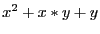HullConsistencyStrong will rewrite the equation as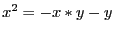while HullConsistency will use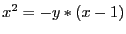which will lead to a better interval evaluation of the left hand term
The _2b procedure must not be used with distance equations as the 2B filtering is already implemented in the solver.Next: Analyzing systems of equations Up: Solving with Interval Analysis Previous: Initial domain and simplification   Contents
Jean-Pierre Merlet 2012-12-20